# Is Potassium hydroxide (KOH) an acid or base? - Strong vs Weak

Home  > Chemistry Article > Is KOH an acid or base?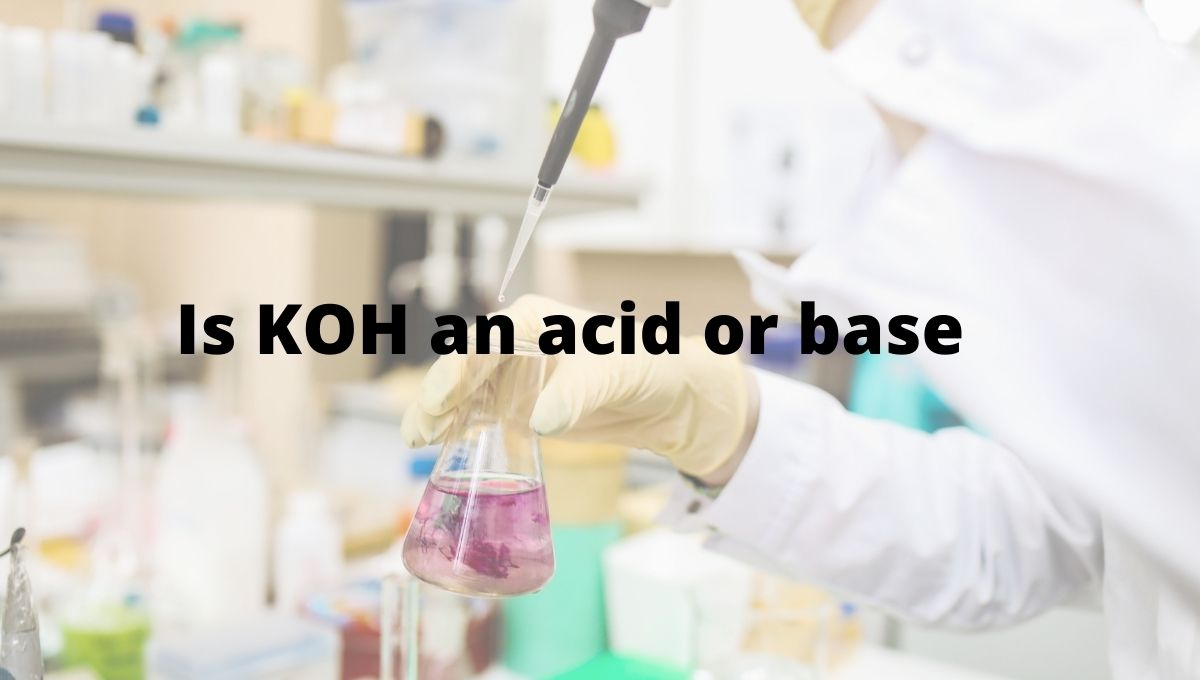Potassium hydroxide is an inorganic compound with the chemical formula KOH. It is a white solid mainly used for manufacturing soft soaps. It is also known as caustic potash or Lye. It can cause severe irritation to the skin and other tissue.

In this article, we will discuss Is Potassium hydroxide (KOH) an acid or base? Is it strong or weak? It’s conjugate pairs, etc.

So, Is KOH an acid or base? KOH is base because when it is dissolved in an aqueous solution it gives two ions K+ and OH. And any molecule which gives OH in a water solution is defined as a base in nature. Its pH value lies between 12-13.

KOH is generally less preferred for industrial uses because of its high-cost issue.

 Name of Molecule Potassium hydroxide Chemical formula KOH Acid or Base? Strong base Conjugate acid K+ pH value 12 to 13 Basicity (pKb) -0.7
Page Contents

## Why KOH is base?

A base is defined as a proton acceptor or lone pair donor. When KOH dissolves in water is split into two ions K+ and OH

As KOH dissociates into K+ and OH, this OH ion accepts the proton (H+) to become water.

⇒ KOH + (aq) → K+ (aq) + OH– (aq)

Theoretically, we have three concepts to check whether KOH is acid or base.

(a). Arrhenius concept

(b). Bronsted-Lowry concept

(c). Lewis concept

Let’s check whether KOH acid or base according to these concepts.

(1). Arrhenius concept:

According to the Arrhenius concept, the compound is said to be base when it produces OH ions through ionization or through dissociation in water and increases the concentration of OH ions in an aqueous solution.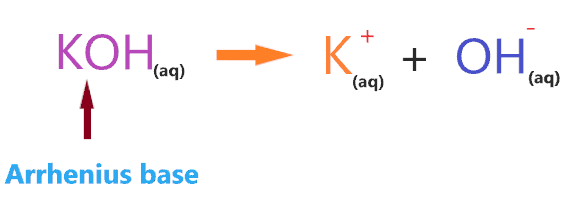Clearly, when KOH reacts with the aqueous solution it produces OH ions, hence,  KOH is an Arrhenius base.

(2). Bronsted-Lowry concept:

According to the Bronsted-Lowry concept, a compound is said to be base when it accepts the proton from other compounds. Or you can say proton acceptor is said to be Bronsted-Lowry base.

Take an example for knowing whether KOH base or acid according to the Bronsted-lowry concept-

Consider the reaction of KOH with HCl.According to the above reaction, KOH has two ions K+ and OH, when they react with HCl then OH accepts H+ from HCl to forms H2O.

So, KOH ion accepting the proton from another compound(HCl), Hence, KOH will act as a Bronsted-Lowry base.

(3). Lewis concept:

Here comes the most important concept of acid and base. According to the lewis concept, a compound is said to be acid when it accepts the pair of electrons and a compound is said to be base when it donates the pair of electrons.

What does it mean?

It means Lewis acid is a compound that has a deficiency of electrons that’s why they are lone pair acceptors and lewis’s base is a compound that is rich in electrons so, they are lone pair donors.

⇒ Lewis acid → lone pair acceptor

⇒ Lewis base → lone pair donator

Remember: Lewis acid is electrophile because they are electron loving and lewis’s base is a nucleophile that hates the electrons.

As KOH contains OH ion that has three lone pairs of electrons and it can easily donate these pairs of electrons to form a covalent bond with another compound acceptor.

So, we can say KOH is lewis’s base also.

## Is Potassium hydroxide(KOH) a strong base or weak base?

To know whether Potassium hydroxide(KOH) is a strong base or weak, you must know the basic difference between a strong base or a weak base.

Strong base: A compound is a strong base when it completely dissociates in an aqueous solution and liberates a large number of hydroxide ions. All moles of the base dissociates to yield hydroxide ion(OH) and no undissociated base should be left in the solution.

Example: Barium hydroxide (Ba(OH)2), Calcium hydroxide (Ca(OH)2), Lithium hydroxide (LiOH), Sodium hydroxide (NaOH), etc.

Weak base: A compound is a weak base when it partially or not completely dissociate in an aqueous solution which means not all moles of the base dissociate in a solution to yield OH ion, and at equilibrium, both undissociated base and their ionized product present in the solution.

Example- Ammonia (NH3), Ammonia hydroxide (NH4OH), Methylamine ( CH3NH2), etc.

 Strong base Weak base They ionize completely. They do not completely ionize. They are highly reactive. They are less reactive compare to a strong base. The value of pH lies between 10 to 14. The value of pH lies between 7 to 10. They have a high equilibrium constant. They have a less equilibrium constant. They are good electrolytes. They are not so good electrolytes compare to a strong base. Example – NaOH, KOH, LiOH, etc. Example – CH3NH2, NH3, NH4OH, etc.

So, Is Potassium hydroxide (KOH) a strong base or a weak base? KOH is the strong base because it completely dissociates in an aqueous solution to give OH ion and no moles of it remain undissociated inside the solution. And the amount of OH ions in an aqueous solution is very high and we know OH ions have a tendency to accept the proton.

So, more proton acceptors present in the solution ultimately make the KOH a strong base.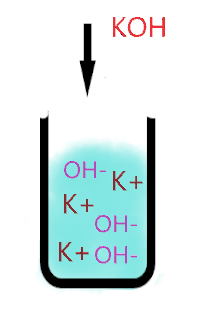As you see in the above aqueous solution when KOH is dissolved in water, it is completely ionized into the ions(K+ and OH). No undissociated molecule(KOH) present in the solution, only ionized ions present everywhere in the solution.

⇒ KOH + (aq) → K+ (aq) + OH– (aq)

The single arrow used in the above reaction shows that only a forward reaction takes place that means, once the KOH molecule breaks into the ions, these ions will not reform with each other to form KOH again.

As K+ is a very weak conjugate acid of KOH, hence it has no ability to react with either OH ion or with water molecules ions. Thus, only splitting ions(K+ and OH) remain in the solution.

Or you can also assume the K+ as a spectator ion because it is almost useless in solution, it has no effect on the pH value of the solution.

“A spectator ion is an ion that does not take part in the chemical reaction and is found in solution both before and after the reaction.”

Hence, a large number of hydroxide ions present in the aqueous solution of KOH, steadily increase the pH value and rises the effect of the basic in solution. And all these make potassium hydroxide a strong base in nature.

Also, the base dissociation constant value(Kb) for KOH is greater than 1.

An base dissociation constant (Kb) is a quantitative measure of the strength of an base in solution.

⇒ If the value of the dissociation constant of the base is greater than 1 (Kb > 1), then the nature of the compound is a strong base.

⇒ If Kb < 1, then the nature of the compound is a weak base.

So, the higher the value of the base dissociation constant, the larger is the strength of a base in solution.

Here’s the list of some common strong/weak acids and bases.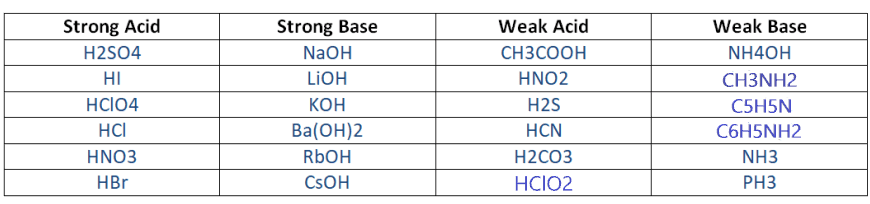## Why Potassium hydroxide(KOH) is not an acid?

Acid is a compound that donates H+ ions when dissolving them in an aqueous solution. It means the acid is the proton donator.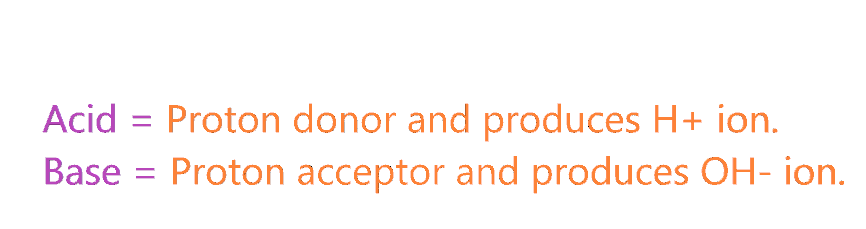So, Is KOH is an acid? KOH is not an acid because when it dissolves in an aqueous solution, it furnishes a large amount of OH ions. And OH ion is a good acceptor of the proton, which means KOH ions are proton acceptors, not a proton donator.

KOH + (aq) → K+ (aq) + OH– (aq)

We have two theories given by Arrhenius and Bronsted-Lowry to check why KOH is not acid?

According to the Arrhenius theory, any compound is said to be acid when it liberates H+ ion in water or aqueous solution. And we know KOH in an aqueous solution only produces OH ion. So, KOH is not an Arrhenius acid.

According to the Bronsted-lowry theory, any compound is said to acid when it has a tendency to donate the proton to other compounds. Let’s take an example to understand this-

⇒ KOH(aq) + HCl(aq) → KCl(aq) +H2O(l)

When KOH reacts with a strong acid (HCl), the OH ion accepts H+ from HCl to produce H2O, which means here KOH act as a proton acceptor.

So, here, KOH is not a Bronsted-lowry acid since it is accepting the proton.

## Why KOH act as a Lewis base?

Lewis’s theory is a very important acid-base theory to check whether a compound (KOH) acid or base?

According to the Lewis theory, a compound is said to be acid when it accepts the pair of electrons and a compound is said to be base when it donates the pair of electrons.

Lewis acid → electron pair acceptor

Lewis base → electron pair donator

Now, why KOH acts as a Lewis base? To know this first we should know what type of compound is Lewis acid and Lewis base.

Lewis acid: They are electron-loving compounds and are also known as electrophilic compounds that accept the electron pair from Lewis bases.

Some examples of Lewis acids that can accept the pair of electrons from another species.

1. The molecules or ions with an incomplete octet of electrons. Example – BF3, BCl3, AlF3, etc.
2. The molecule that central atom has an empty d-orbital. Example- SiCl4 (1s22s22p63s23p3d0).
3. The compounds that the central atom formed multiple bonds with the adjacent atoms. Example – SiO2 (O = Si = O), CO2, SO2, etc.
4. Simple cations like H+, Na+, Mg+2, Al+3, etc. are lewis acids since they are able to accept the electrons. ( Exception NH4+, PH4+ are not a lewis acid)

Lewis base: They are electron-rich species and also known as nucleophile compounds that donate the electron pairs to the Lewis acids.

Some examples of Lewis bases that can donate the pair of electrons to other species.

1. The negative ion such as H, OH, F, etc.
2. Species that have lone pair of electrons. Example – NH3, H2O, CH3, etc.

Let’s take an example to understand why KOH acts as Lewis base?

⇒ KOH + HCl → KCl + H2O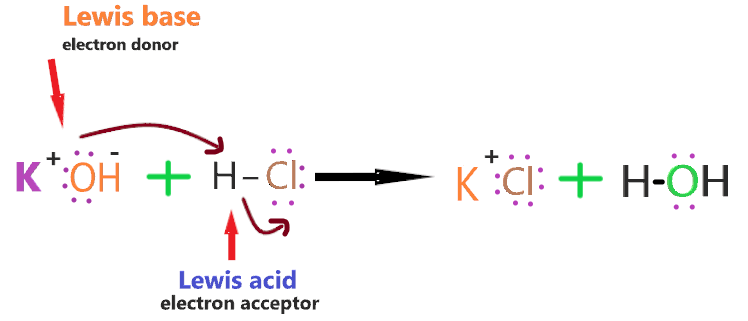As we know, In the HCl molecule, the chlorine atom is more electronegative than the hydrogen atom, therefore, more electrons will be attracted to the side of the chlorine atom, which will induce a negative charge on the chlorine atom and a slightly positive charge on the hydrogen atom.

Now, in the KOH compound, the oxygen atom has lone pair that is attracted towards the slightly positive hydrogen atom in the HCl molecule.

As the lone pair on the oxygen atom approaches the hydrogen atom, the electrons in the HCl bond are repelled still further towards the chlorine atom.

And in this process, a coordinate bond is formed between the hydrogen and hydroxyl group, and the chlorine breaks away.

Therefore, the HCl molecule will act as a Lewis acid as it accepting the pair of electrons from KOH and, in this process, it breaks up. [Source google: Lewis acid & base]

So, HCl accepts the lone pair of the electron, therefore, it is Lewis acid and KOH donates the lone pair of the electron, therefore, it is Lewis base.

## What is the conjugate acid of KOH?

In technical terms, Compounds differentiated from each other by a single proton(H+) are said to be Conjugate acid-base pairs.

In simple terms, when the proton is removed from parent acid then the compound is formed which is called the conjugate base of that acid and when the proton is added to the parent base then the compound is formed which is called conjugate acid of that base.

Concept of Conjugate acid-base pair-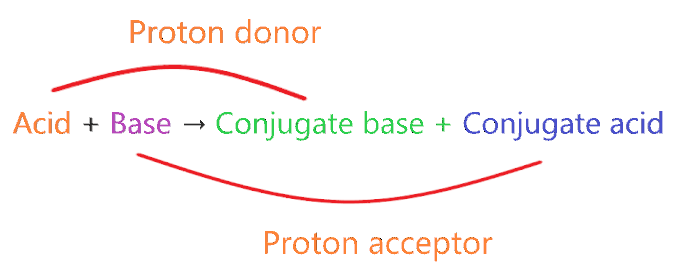• A very weak acid forms the strong conjugate base.
• A very weak base forms strong conjugate acid.
• A very strong acid forms the weak conjugate base.
• A very strong base forms weak conjugate acid.

⇒ Very weak means it doesn’t act as acid or base when dissolved in an aqueous solution.

⇒ Very strong means, acid or base ionizes 100% when dissolved in an aqueous solution.

Example 1: NH3 + H+ → NH4+

Here in this reaction, NH3 is a weak base and we know a weak base always forms a conjugate acid(not necessarily the strong one).

So, NH4is the conjugate acid of NH3.

Example 2: HCl → H+ Cl

In this reaction, HCl is a very strong acid and we know very strong acid always forms the weak conjugate base by donating one proton.

So, Cl is the weak conjugate base of HCl.

Example 3: CH3COOH + H2O → CH3COO + H3O+

Here in this reaction, CH3COOH is a weak acid that donates the proton to form CH3COO, which means CH3COO– is the conjugate base of CH3COOH.

H2O behaves as the base because it accepts the proton from CH3COOH to form H3O+, which means H3O+ is the conjugate acid of H2O.

⇒ The Conjugate acid of KOH is K+. [Check here–]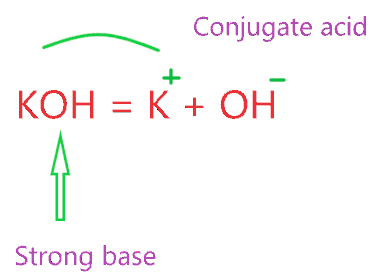Note: The conjugate acid of KOH can be also H2O if you assume K+ as a spectator ion in the aqueous solution because it has nothing to do in the solution since it doesn’t hydrolyze.

So, only OH ion is left and conjugate acid is formed when H+ is added.

Therefore, OH + H+ = H2O is the conjugate acid of the hydroxide ion(OH).

## How to know if KOH is acid or base practically?

Till now we learn how to know if compound acid or not theoretically. To know practically, one of the easiest ways to use litmus paper.

Litmus is a water-soluble mixture of different dyes extracted from lichens. It is often absorbed onto filter paper to produce one of the oldest forms of pH indicator, used to test materials for acidity. [Wikip-]

Note: When Red litmus paper turns blue then the compound is said to be base. And when blue litmus paper turns red then the compound is said to be acidic.

When KOH is contacted with red litmus paper then litmus paper turns into blue color. So, we can say KOH is the base.

## Is KOH alkali or not?

Alkali is a strong base that produces hydroxide ions when it is dissolved in water. All soluble hydroxides like lithium, cesium, sodium, potassium, etc. are alkali metals.

An alkali is said to be strongest when it produces almost all OH- ions when it is dissolved in water.

As for KOH when dissolving in water it produces almost all OH- ions that ultimately make it strong alkali.

## Uses of Potassium hydroxide

• It is used in manufacturing soft soaps.
• In the food industry, it is used as a food thickener, stabilizer, and pH controlling agent.
• It is used as an electrolyte in alkaline batteries.
• It is used in the manufacturing of biodiesel.

## Conclusion

I hope you must enjoy this article on Is Potassium hydroxide(KOH) is an acid or base? And I think this article clears all of the doubt you have over Why KOH is base, why it’s not an acid, is its a strong base or not, etc. Still, if you have a doubt regarding this then you must clear it by asking in a comment box. Lastly, I am giving you a short overview of this article.

• Is KOH an acid or base? Potassium hydroxide (KOH) is the base since it releases OH ions on dissolving in an aqueous solution.
• KOH is the strong base because it completely dissociates in an aqueous solution and produces a large number of OH ions.
• We have three theories to check whether KOH base or acid. (a). Arrhenius, Bronsted-Lowry, Lewis acid-base theory, etc.
• KOH act as a Lewis base as it has an OH ion which can donate the electron pair to another compound acceptor.
• KOH is a strong base that forms weak conjugate acid.
##### Subscribe to Blog via Email

Join 2 other subscribers

Share it...